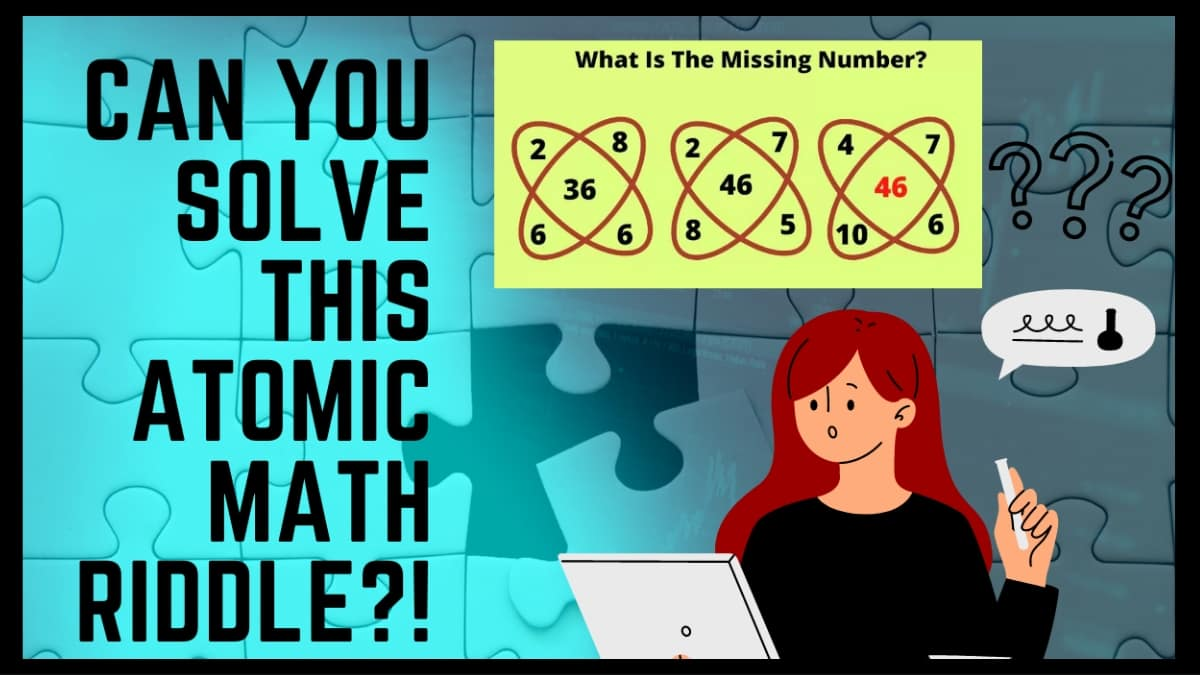# Math Riddles: Can You Solve This Atomic Math Puzzle And Find The Missing Number? Test Your IQ Here

Math Riddles are exercises designed to stimulate cognitive function, encouraging the brain to work to complete the exercise. Solve this atomic math puzzle and find the missing number. You can test your IQ here!Math Riddles: Can You Solve This Atomic Math Puzzle And Find The Missing Number? Test Your IQ Here

Math Riddles are exercises designed to stimulate cognitive function, encouraging the brain to work to complete the exercise.

While many people like to do Math Riddles for fun, these simple exercises have other uses as well like for instance neurologists, for example, often utilize them when they work with patients to arrive at a diagnosis, and teachers often use them in the classroom as educational tools.

 Math Riddle Fact! Some studies also suggest that doing Math puzzles helps keep the mind fit. In older adults, people who do Math Riddles tend to perform better on various tasks related to cognitive function.

Build your mind and brain power with this Math Riddle!

Those who are good at math will be able to solve this math puzzle with ease but anyone with a sharp mind and a penchant for mathematical equations and reasoning can solve this math puzzle.

So let’s go!

Math Riddle Challenge:  What Is The Missing Number? To solve a mathematical Puzzle, Observe the mathematical puzzle and try to solve it and find the solution for the problem.

Easy?

Let's set a timer for you!

Let's see if you can find the number in 30 Seconds!

tick..

tock..

tick..

3..

2..

1..

TIME'S UP!

Could you do it?

If you could not find the solution, we have one for you.

## Let's Solve this Together

If you are still working to figure it out, we have the solution to this mathematical puzzle. This puzzle will allow you to see how keen you are and how reasonable an observer you are. If you still need help, the picture below will help you understand the solution.

As you can see that all figures have the same logic

if we compare the First Figure and the Second Figure then:

## First Figure

### 48-12= 36

as shown in the figure the middle number is 36 if we multiply the corner numbers and subtract their sum.

## Second Figure

### 56-10=46

hence, both the figures have the same logic and now we can check for the third figure.

## Third Figure

we will apply the same logic to find the missing number.

Great Work!